## Laplace Properties:

Laplace transforms have the following Laplace Properties.

#### (a) Superposition Property:

The first Laplace Properties is Superposition Property. The Laplace transform of the sum of the two or more functions is equal to the sum of transforms of the individual function,

i.e. if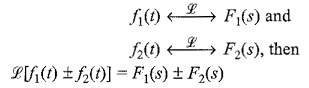Consider two functions f1(t) and f2(t). The Laplace transform of the sum or difference of these two functions is given by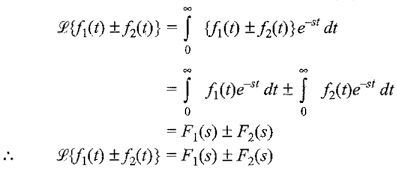#### (b)Linearity property:

The first Laplace Properties is Linearity Property. If K is a constant, then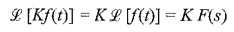Consider a function f(t) multiplied by a constant K. The Laplace transform of this function is given by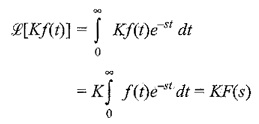If we can use these two properties jointly, we have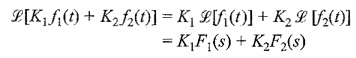### Laplace Transform of Some Useful Functions:

(i) The unit step function f(t) = u(t)

where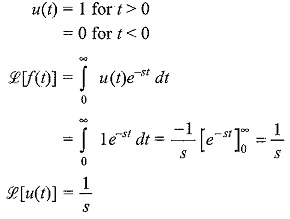(ii) Exponential function f(t) = e-at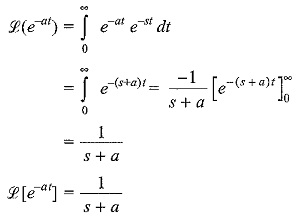(iii) The cosine function: cos ωt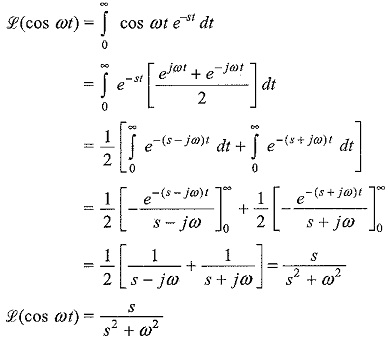(iv) The sine function: sin ωt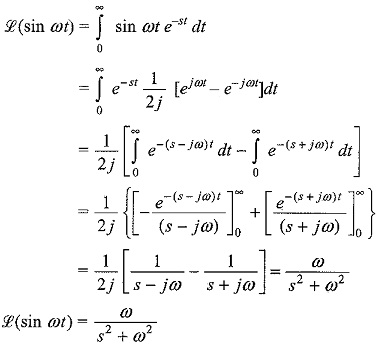(v) The function tn, where n is a positive integer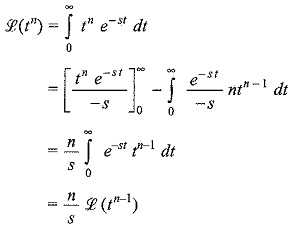Similarly,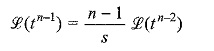By taking Laplace transformations of tn-2, tn-3,…. and substituting in the above equation, we get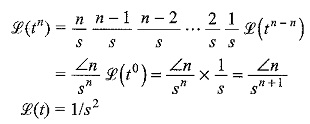(vi) The hyperbolic sine and cosine function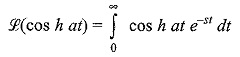Similarly,

Scroll to Top